Search

Minors and Cofactors: Introduction;
Expanding Along a Row
(page 1 of 3)Finding the determinant of a 2×2 matrix is easy: You just do the criss-cross multiplication, and subtract: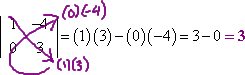The process for 3×3 matrices, while a bit messier, is still pretty straightforward: You add repeats of the first and second columns to the end of the determinant, multiply along all the diagonals, and add and subtract according to the rule: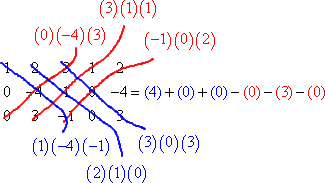But for 4×4's and bigger determinants, you have to drop back down to the smaller 2×2 and 3×3 determinants by using things called "minors" and "cofactors".

A "minor" is the determinant of the square matrix formed by deleting one row and one column from some larger square matrix. Since there are lots of rows and columns in the original matrix, you can make lots of minors from it. These minors are labelled according to the row and column you deleted. So if you were to go, say, to the a2,4 entry from some matrix A and cross out the row and column that pass through that entry (that is, if you remove the second row and the fourth column of the matrix), the determinant of the new (and smaller) matrix is called "the minor M2,4".

 the matrix A cross out all entries sharing arow or column with entry a2,4 the minor M2,4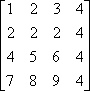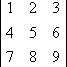Once you find a minor Mi, j, you take the subscript on the name of the minor (the "i, j" part) and add the two numbers i and j. Whatever result you get from this addition, make this value the power on 1, so you get "+1" or "1", depending on whether i + j is even or odd. Then multiply this on the minor
Mi, j. This gives you the "cofactor" Ai, j. That is:

(1)i+j  Mi, j  =  Ai, j

You're still not done though. Yes, there's more.

To find the determinant of the matrix A, you have to pick a row or a column of the matrix, find all the cofactors for that row or column, multiply each cofactor by its matrix entry, and then add all the values you've gotten.   Copyright © Elizabeth Stapel 2006-2011 All Rights Reserved

Okay, yeah; that probably didn't make much sense. Here's another way of saying it:

• You've got some matrix A. You need to find the determinant of it.
• It's too big to find by the simpler methods, so you'll have to find it by "expanding along a row or column".
• Your first step in this "expanding" will be picking a row or a column. Let's say you pick the third row.
• For each entry in the third row, you will find the cofactor of that entry and multiply the entry by its cofactor. That is, for the a3,1 entry of A, you will find the cofactor A3,1, and then you'll multiply the cofactor by the a3,1 entry: (a3,1)(A3,1). For entry a3,2, you will find the cofactor A3,2, and multiply: (a3,2)(A3,2). And so forth.
• Then you will add up all of these products: (a3,1)(A3,1) + (a3,2)(A3,2) + (a3,3)(A3,3) + ....

The resulting sum is the value of the determinant of the matrix A.

(The above mess is why nobody does determinants by hand if it can be avoided: there's just so much error-prone mindless grunt-work involved.)

Weird fact: It doesn't matter which row or column you use for your expansion; you'll get the same value regardless. But this flexibility can be useful.

• Find the determinant of the following matrix by expanding (a) along the first row and (b) along the third column. (c) Compare the results of each expansion.
•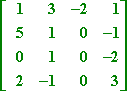(a) To expand along the first row, I need to find the minors and then the cofactors of the first-row entries: a1,1, a1,2, a1,3, and a1,4.M1,1 M1,2 M1,3 M1,4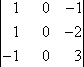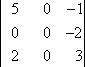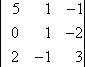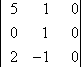M1,1 = 0 M1,2 = 0 M1,3 = 3 M1,4 = 0 A1,1 = (1)1+1M1,1  = (1)2(0)= 0 A1,2 = (1)1+2M1,2  = (1)3(0)= 0 A1,3 = (1)1+3M1,3 = (1)4(3)= 3 A1,4 = (1)1+4M1,4  = (1)5(0)= 0

So the determinant of this matrix is:

det(A) = a1,1A1,1 + a1,2A1,2 + a1,3A1,3 + a1,4A1,4
= 1(0) + 3(0) + (2)(3) + 1(0)

= 6

Now I need to expand down the column...

Top  |  1 | 2 | 3  |  Return to Index  Next >>

 Cite this article as: Stapel, Elizabeth. "Minors and Cofactors: Introduction; Expanding Along a Row."     Purplemath. Available from https://www.purplemath.com/modules/minors.htm.     Accessed [Date] [Month] 2016

Study Skills Survey

Tutoring from Purplemath
Find a local math tutor